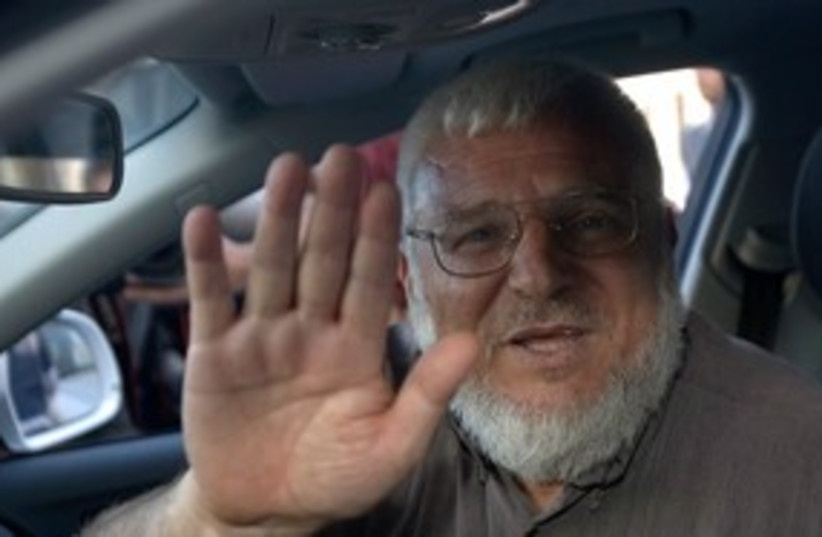(function (a, d, o, r, i, c, u, p, w, m) { m = d.getElementsByTagName(o), a[c] = a[c] || {}, a[c].trigger = a[c].trigger || function () { (a[c].trigger.arg = a[c].trigger.arg || []).push(arguments)}, a[c].on = a[c].on || function () {(a[c].on.arg = a[c].on.arg || []).push(arguments)}, a[c].off = a[c].off || function () {(a[c].off.arg = a[c].off.arg || []).push(arguments) }, w = d.createElement(o), w.id = i, w.src = r, w.async = 1, w.setAttribute(p, u), m.parentNode.insertBefore(w, m), w = null} )(window, document, "script", "https://95662602.adoric-om.com/adoric.js", "Adoric_Script", "adoric","9cc40a7455aa779b8031bd738f77ccf1", "data-key");
var domain=window.location.hostname; var params_totm = ""; (new URLSearchParams(window.location.search)).forEach(function(value, key) {if (key.startsWith('totm')) { params_totm = params_totm +"&"+key.replace('totm','')+"="+value}}); var rand=Math.floor(10*Math.random()); var script=document.createElement("script"); script.src=`https://stag-core.tfla.xyz/pre_onetag?pub_id=34&domain=\${domain}&rand=\${rand}&min_ugl=0\${params_totm}`; document.head.append(script);

## Abdel Aziz Dweik says Syrian regime is a "stab in the heart," removal of Assad would boost Palestinian cause.Hamas official Aziz Dweik 311 (R)